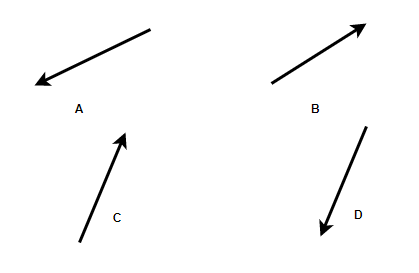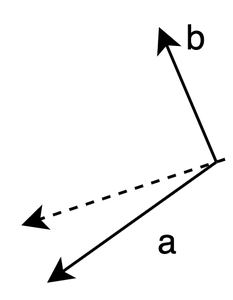Open in App
Not now

# Vector Algebra

• Last Updated : 16 Jun, 2021

Often while searching for someplace, people ask for directions. The usual answer is to go in a particular and for some distance. So, it can be concluded that only direction is not enough, the magnitude of the distance to be traveled in that direction is also equally important. In mathematical terms, this information about the direction and the magnitude is usually conveyed through vectors. Vectors are very useful in the field of game design and artificial intelligence. Let’s study the concept of vector in detail.

### Introduction to Vectors

In physics and mathematics, we frequently come across several types of quantities. All these quantities can be classified into two major categories — scalar quantities and vector quantities. Consider an example – the height and weight of a person can be described by a single number like 75 Kg or 150cm. These quantities only have magnitude, they do not require any extra information. Such quantities are called scalar quantities. Now, let’s consider another situation, for example, the coach of the football team wants to teach his goalkeeper to pass the ball to another player, now he will have to describe towards the region he should send the ball (Direction) and how hard he should hit (magnitude). This quantity requires both magnitude and direction. Such quantities are called vectors.

A vector is a quantity which has a direction and a magnitude.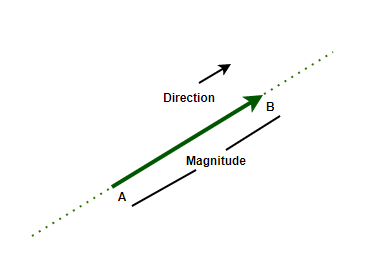In the above figure, the length of the line shows the magnitude of the vector, and the arrowhead points to its direction. It is basically a directed line segment. Its starting point A is called the initial point and point B where it ends is called the terminal point.

### Types of Vectors

Vectors can be classified into different categories on the basis of their magnitude and direction:

1. Zero Vector: A vector whose initial and terminal points coincide, is called a zero vector. It cannot be assigned any direction or magnitude.
2. Unit Vector: A vector that has a unit magnitude. A unit vector in the direction of given vectoris denoted by.
3. Co-initial Vectors: The vectors which start from the same point are called co-initial vectors.
4. Collinear Vectors: Two vectors are said to collinear if they are parallel to the same line, irrespective of their magnitudes and direction.
5. Equal Vector: Two vectors are considered equal if they have the same direction and magnitude.
6. Negative of a Vector: It is a vector whose magnitude is the same, but the direction is opposite to that of a vector.

A vectorcan be thought of as a displacement from point A to point B. To understand the need for addition and subtraction of vectors, consider an example. In the figure, Laxmi goes from point A to point B and then to point C following the vectors shown in the figure. Now, after reaching point C, the net displacement made by this girl is from A to C which is given by the vector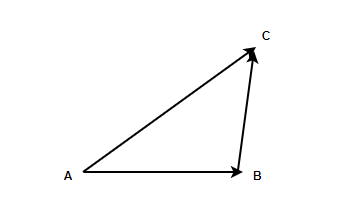This can be expressed in terms of vectors as,=This is known as the triangle law of vector addition. In general, for two vectorsandtheir addition is done such that the initial point of one vector coincides with the terminal points of the other.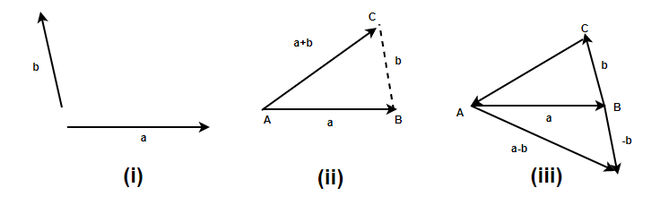In the above figure, we have two vectors a and b given in part(i), in the part(ii) the vector b is shifted without changing its direction and magnitude such that now the initial point of vectorlies on the endpoint of vector a. In the case of subtraction, the direction of vector b is reverse and then both vectors are added.

Addition and difference have almost similar concepts and methods, except that while calculating the difference the direction of one vector is reversed.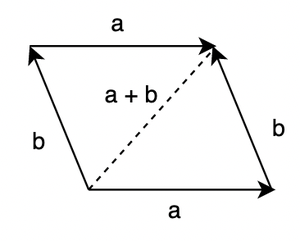If those two vectors are represented as adjacent sides of parallelograms, then their sum in magnitude and direction represents the diagonal of the parallelogram. This is known as the parallelogram law of vector addition.

Property 1: Vector addition follows commutative property. For two vectorsand.### Multiplication of Vectors with a scalar

Let’s sayis a given vector and “k” be a scalar. The product of the scalar will either increase or decrease the magnitude of the vector. The direction of the vector will remain the same. The increase or decrease in the magnitude of the vector will depend upon the value of the scalar that is multiplied by the vector. The figure below shows the vector A multiplied by some scalar quantity. Notice how the length of the vector changes after being multiplied by a scalar.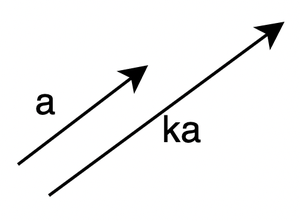The magnitude of the vector will become,When k = -1, the direction of the vector is reversed,This is called the additive inverse of the vector.

Let’s see some sample problems on these concepts

### Sample Problems

Question 1: Out of the following quantities, find the quantities which are vectors:

1. Weight
2. Force
3. Velocity
4. Height

1. Weight does not need directions to be expressed. Hence, it’s a scalar quantity.

2. Force needs the direction to where it must be applied. Thus, it’s a vector quantity.

3. Velocity also has direction. It is also a vector quantity.

4. Height can be simply expressed as a number, there is no need to give direction. Thus, it’s a scalar quantity.

So, Only Force and Velocity are vectors.

Question 2: State whether the following statement is True or False.

“Collinear are vector are those vectors which start from the same point”

This state is False.

According to the definition mentioned above,

“Two vectors are said to collinear if they are parallel to the same line, irrespective of their magnitudes and direction.”

They need to be parallel to same line. It is not necessary that the vectors which start from same point are parallel to same line.

Figure

Question 3: The figure below shows four vectors,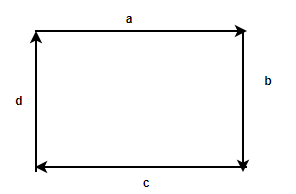State true or false based on this figure.

1.andare collinear
2.andare co-initial vectors.

(i)andare collinear

Collinear vectors are parallel to the same line, these vectors are not parallel to each other. They cannot be parallel to the same line. Thus, false.

(ii)andare co-initial vectors.anddon’t have the same initial points. Thus are not co-initial vectors.

Question 4: Represent graphically, 40Km displacement 45° east to the North.

Solution:

The value of the displacement is 40Km. Let’s say this point is represented byMagnitude of this vector is 40Km.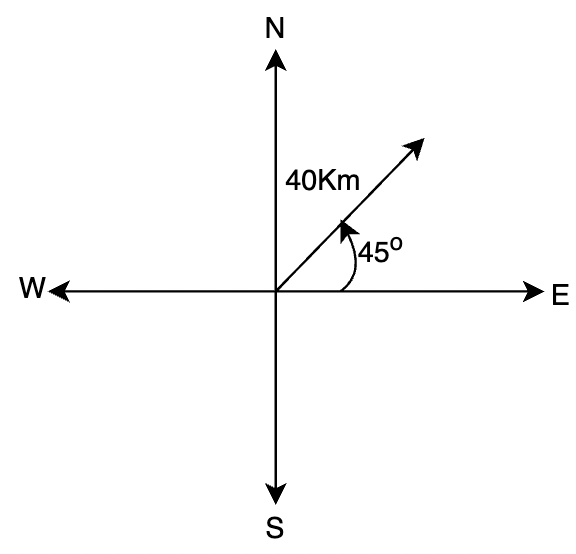Question 5: Let’s say two vectors are definedandSimplify and FindSolution:

Given,….(1)….(2)

Goal is to findSubstituting the values of these vectors from equation (1) and (2)Question 6: For the given vectors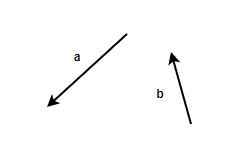Choose which of the following best represents the